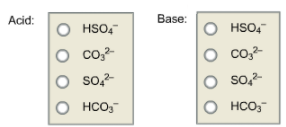Chemistry Practice Problems Conjugate Acids and Bases Practice Problems Solution: Identify all the acids and bases in the following ...

🤓 Based on our data, we think this question is relevant for Professor Pinkston's class at UNR.

# Solution: Identify all the acids and bases in the following reaction.  HSO4-(aq) + CO32- (aq) ⇌ SO42- (aq)+ HCO3-(aq).

###### Problem

Identify all the acids and bases in the following reaction.

HSO4-(aq) + CO32- (aq) ⇌ SO42- (aq)+ HCO3-(aq).Conjugate Acids and Bases

Conjugate Acids and Bases

#### Q. What is the conjugate base of HSO 3− and HPO22−?Express your answer as a chemical formula.

Solved • Fri Feb 16 2018 10:25:26 GMT-0500 (EST)

Conjugate Acids and Bases

#### Q. Give the conjugate acid and base of HSO3-?a) H3SO3, SO32-b) HSO3-,HSO3-c) H2SO3, SO32-d) SO32-, H2SO3e) HSO3, SO32-

Solved • Thu Feb 15 2018 17:51:30 GMT-0500 (EST)

Conjugate Acids and Bases

#### Q. What is the conjugate acid of NH 3? What is the conjugate base of HSO4- ?

Solved • Wed Jan 03 2018 13:07:59 GMT-0500 (EST)

Conjugate Acids and Bases

#### Q. Acetic acid, HC2H3O2, is a weak acid that is in equilibrium in the following given reaction: HC2H3O2(aq) + H2O(l) ⇌ H3O+(aq) + CH3CO2-(aq) a. Identi...

Solved • Thu Dec 14 2017 14:25:49 GMT-0500 (EST)